## Figures index

#### Usama H. Hegazy

American Journal of Mechanical Engineering. 2014, 2(7), 316-327 doi:10.12691/ajme-2-7-31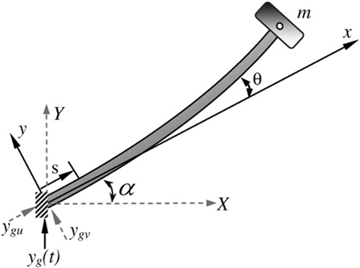• Figure 1. The considered model of a cantilever beam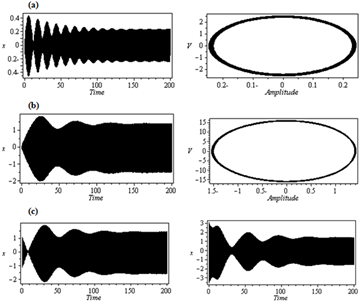• Figure 2. Numerical solution of the cantilever beam: (a) Non-resonant time response solution, (b) Primary resonant solution, (c) Effect of initial conditions on system behavior at primary resonance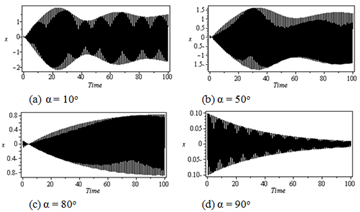• Figure 3. Effect of the orientation angle under primary resonance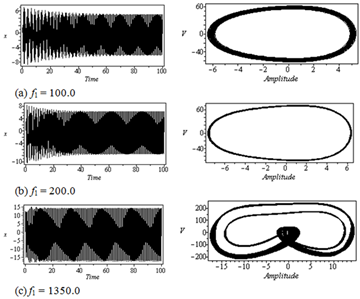• Figure 4. Effect of the external excitation under primary resonance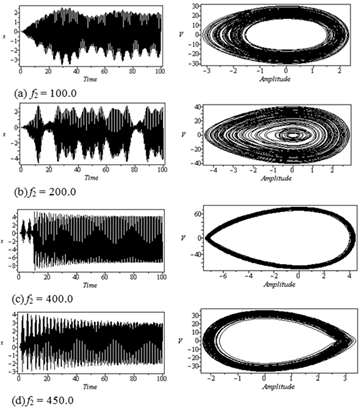• Figure 5. Effect of the parametric excitation under primary resonance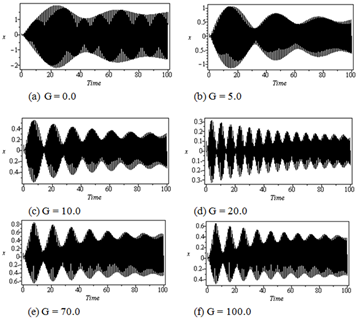• Figure 6. Performance of LPF controller, (a)-(d), and QPF controller, (e) and (f), for different values of the gain when the system subjected to primary resonance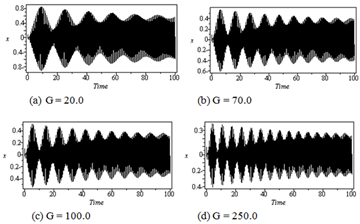• Figure 7. Performance of CPF controller for different values of the gain when the system subjected to primary resonance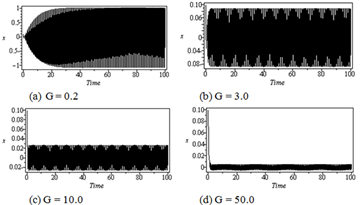• Figure 8. Performance of LVF controller for different values of the gain when the system subjected to primary resonance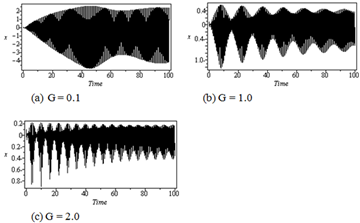• Figure 9. Performance of QVF controller for different values of the gain when the system subjected to primary resonance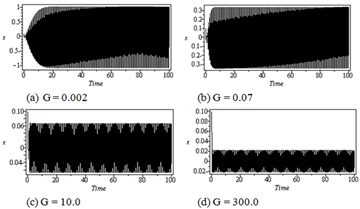• Figure 10. Performance of CVF controller for different values of the gain when the system subjected to primary resonance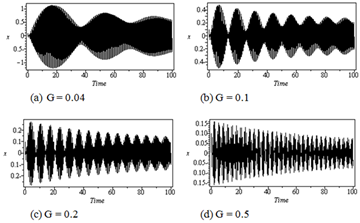• Figure 11. Performance of negative acceleration feedback controller for different values of the gain when the system subjected to primary resonance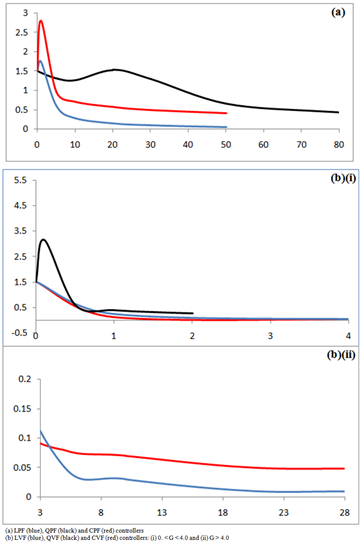• Figure 12. Effect of the gain for various control laws at primary resonance - numerical integration of the system: (x-axis: the gain, y-axis: x-amplitude)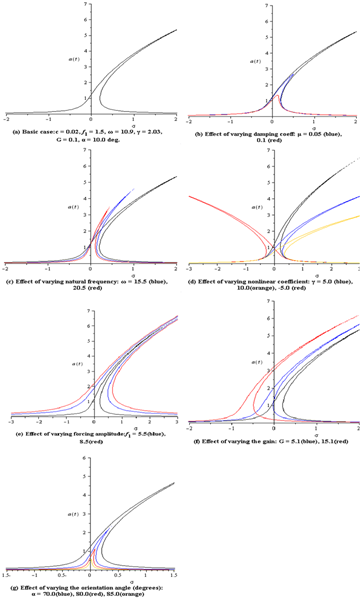• Figure 13. Primary resonant frequency response curve with LPF controller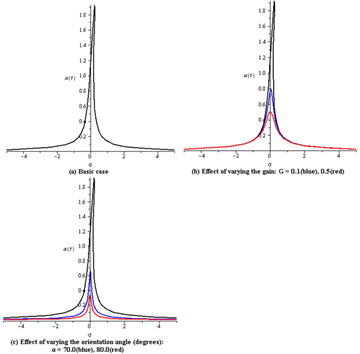• Figure 14. Primary resonant frequency response curve with LVF controller